# README.md In autoshiny: Automatic Transformation of an 'R' Function into a 'shiny' App

autoshiny – R package for automatic transformation of an R function into a Shiny app ================ Aleksander Rutkowski 2018-06-14

## Installation

``````# if package `devtools` not installed, first do this:
# install.packages('devtools')
devtools::install_github('alekrutkowski/autoshiny')
library(autoshiny)
``````

## Key info

There are two key twin functions: `makeApp` and `makeFiles`. Both of them take a function as their first argument/parameter. Function `makeApp` returns a Shiny app object. `makeFiles` produces `ui.R` and `server.R` files. These files can be further edited to tweak the app if needed.

Using autoshiny does not imply any run-time dependency of the compiled app on autoshiny i.e. autoshiny is needed only at the compile time. autoshiny uses standard Shiny input and output widgets and render functions. Tiny helper functions are embedded in the compiled app code to make it self-contained.

All the arguments/parameters of the function passed to `makeApp` and `makeFiles` must have default values which will be used by autoshiny to define each argument’s:

• type/class – Shiny input widget type,
• allowed values – Shiny input widget’s allowed states,
• pre-selected/start-up value of the Shiny input widget – for categorical values (e.g. integers, factors, character vectors that will be the first element of the vector).

The default values of the function arguments/parameters will be also used to pre-evaluate that function in order to test it and to determine the type of its output (return value / side effect) and, hence, the Shiny output widget.

## Examples

``````library(shiny)
``````

### Example 1: Trivial anonymous function

``````makeApp(function(x=1:3, y=5:9) x+y)
``````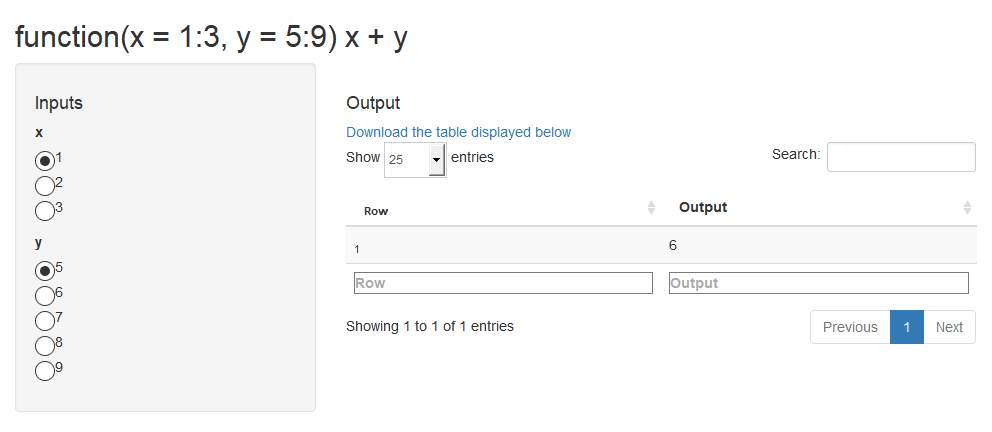See the source code generated with `makeFiles` (and formatted with rfmt) of the app whose initial-state screenshot is displayed above.

### Example 2: Nicer function and argument names

```````Histogram for normal distribution` <-
function(`Number of observations` =
as.integer(c(100,10,1000))) # as.integer => the argument interpreted as categorical
plot(hist(rnorm(`Number of observations`))) # Generic R plots as "return values" are supported

makeApp(`Histogram for normal distribution`)
``````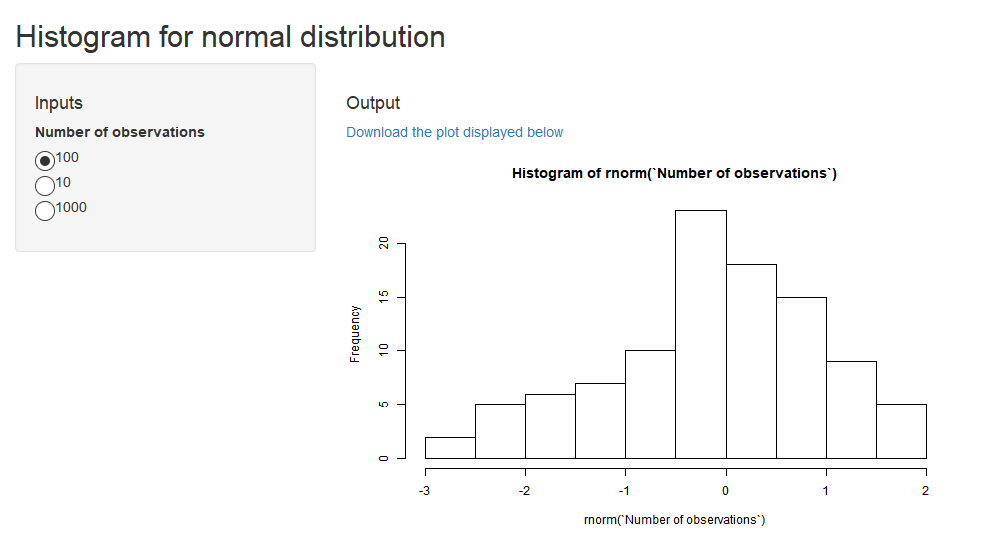See the source code generated with `makeFiles` (and formatted with rfmt) of the app whose initial-state screenshot is displayed above.

```````Table of sin and cos values` <-
function(`Upload CSV file with column "x"` =
data.frame(x = seq(0, 2*pi, .25))) {
dta <- `Upload CSV file with column "x"`
data.frame(X = dta\$x,
`Sin of X` = sin(dta\$x),
`Cos of X` = cos(dta\$x),
check.names = FALSE)
}
makeApp(`Table of sin and cos values`)
``````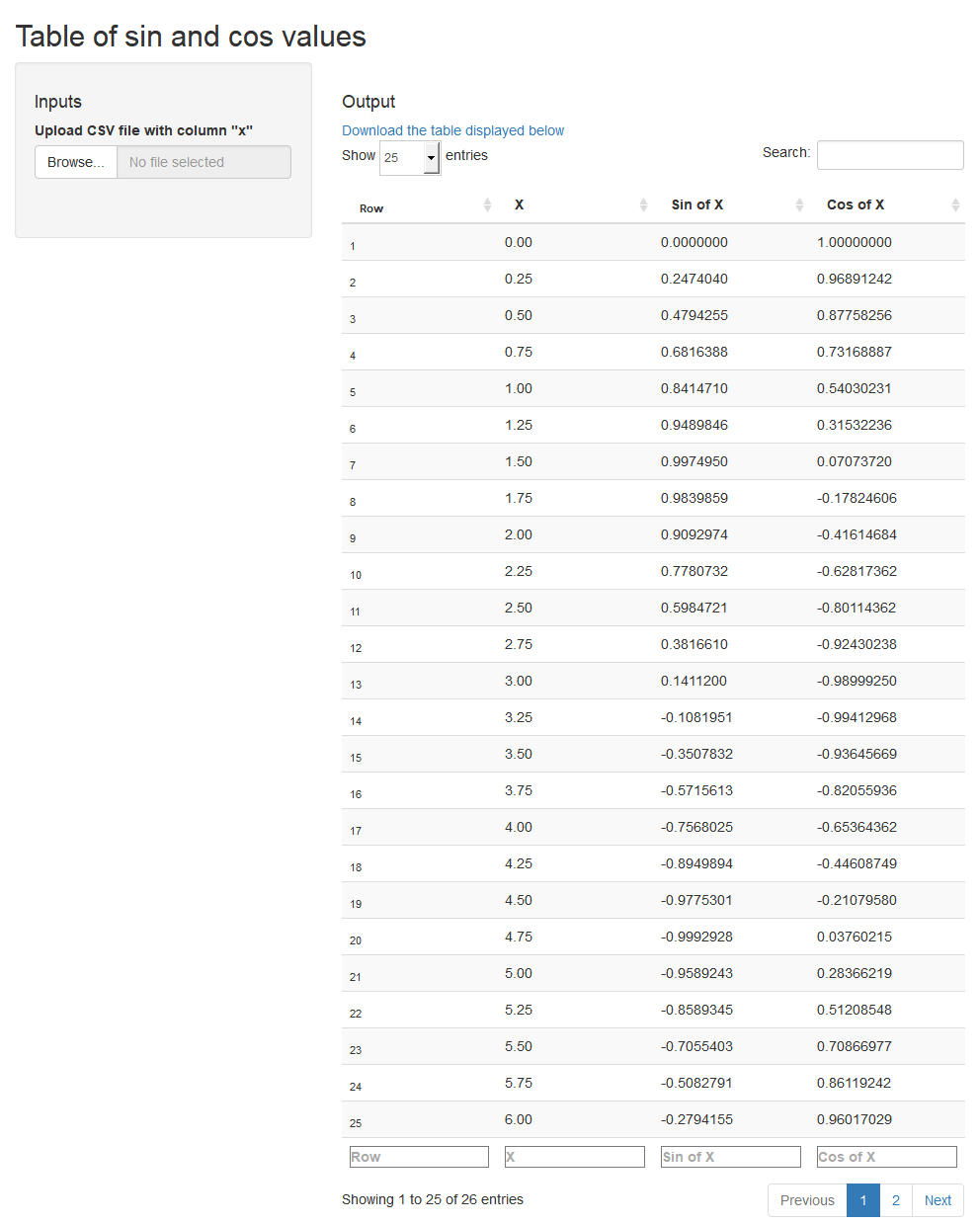See the source code generated with `makeFiles` (and formatted with rfmt) of the app whose initial-state screenshot is displayed above.

### Example 4: Arbitrary input and output files

``````openxlsx::write.xlsx(data.frame(x=1:5,
y=11:15),
'my_test_file.xlsx')
`Excel file in and out` <-
function(`Input Excel file` =
File('my_test_file.xlsx')) { # File() obligatory here!
my.data2 <- within(my.data,
z <- x + y)
openxlsx::write.xlsx(my.data2,
'my_test_file_2.xlsx')
File('my_test_file_2.xlsx') # File() obligatory here too!
}
makeApp(`Excel file in and out`)
``````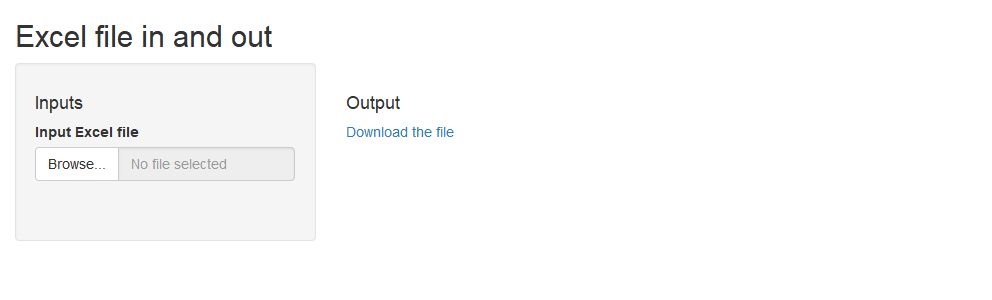See the source code generated with `makeFiles` (and formatted with rfmt) of the app whose initial-state screenshot is displayed above.

### Example 5: Using a button as a (re-)evaluation trigger

Use this option if:

• the evaluation of your functon takes time, so it should not be re-evaluated with every minor change of the value of inputs/arguments/parameter;
• the function is impure e.g. depends on some external data fetched internally and takes no arguments/parameters – in such a case the function would be re-evaluated only through page refresh of the browser; the button is a faster and a more elegant solution.
```````Get "GDP and main components" from Eurostat` <-
function() {
# Getting data from
x <- eurodata::importData('nama_10_gdp')
}
makeApp(`Get "GDP and main components" from Eurostat`,
withGoButton = TRUE)
``````See the source code generated with `makeFiles` (and formatted with rfmt) of the app whose initial-state screenshot is displayed above.

### Example 6: Lists of inputs (arguments) and the output list (composite return value) are always decomposed

```````A function with lists everywhere` <-
function(`First argument group,` = list(`number one` = 1:3,
`number two` = letters[1:3]),
`2nd arg group,` = list(`1st argument` = 11:14,
`second arg.` = LETTERS[1:5]))
list(`Some text` =
as.character(c(`First argument group,`\$`number two`,
`2nd arg group,`\$`second arg.`)),
`Some numbers` =
`First argument group,`\$`number one` +
`2nd arg group,`\$`1st argument`,
`Even a ggplot2 chart` =
ggplot2::qplot(a,b,data=data.frame(a=1:20,b=log(1:20))))
makeApp(`A function with lists everywhere`)
``````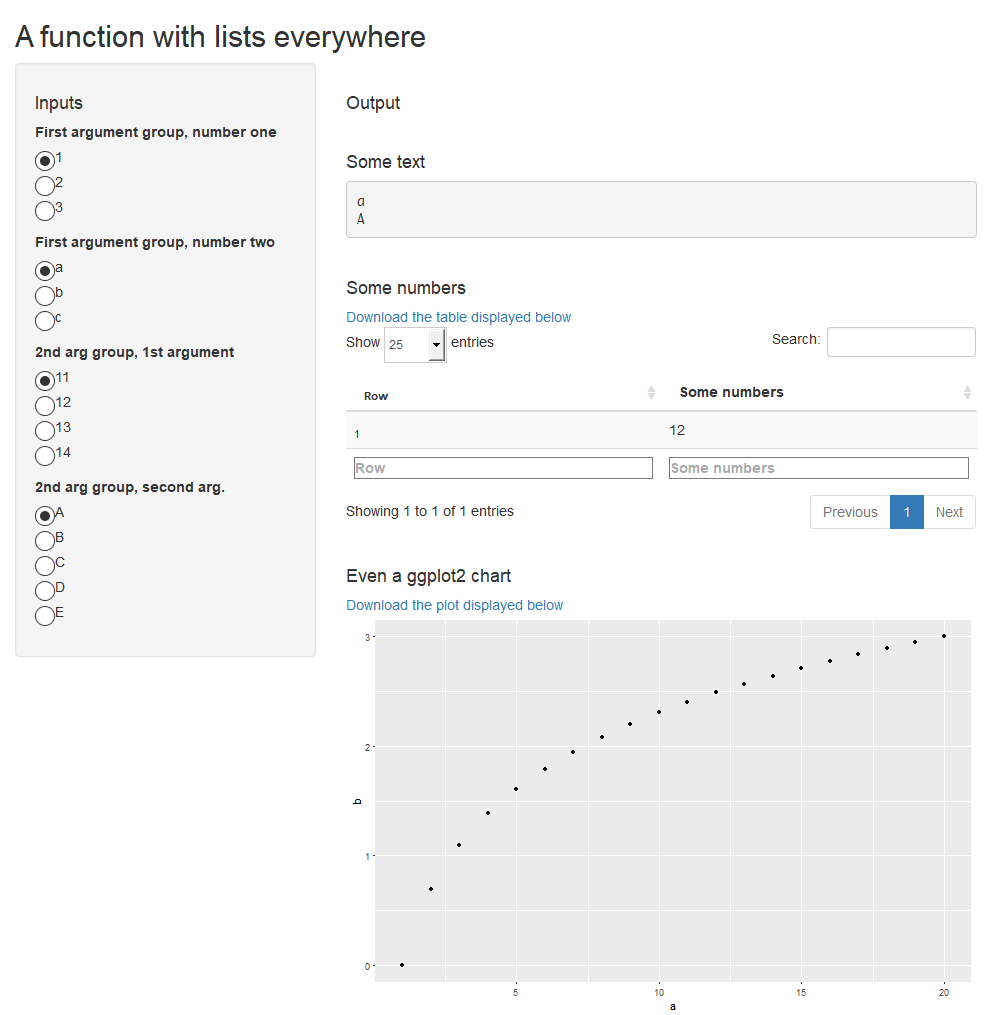See the source code generated with `makeFiles` (and formatted with rfmt) of the app whose initial-state screenshot is displayed above.

## Try the autoshiny package in your browser

Any scripts or data that you put into this service are public.

autoshiny documentation built on May 2, 2019, 1:08 p.m.